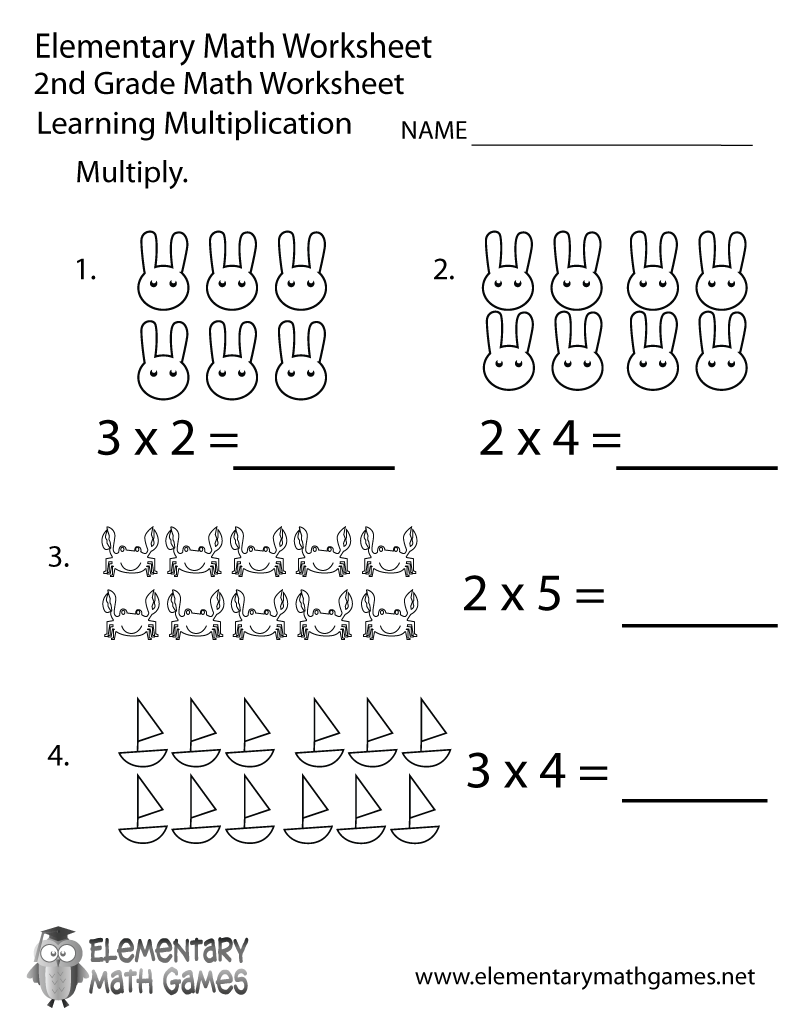# Second Grade Multiplication Worksheet## Learn and Practice Multiplication

This second grade math worksheet is a great way for kids to learn how to multiply. The answers to the multiplication problems are displayed as pictures. The images will help educators explain to second graders how the correct results were achieved.

Here are the two versions of this free second grade math worksheet: